# RS Aggarwal Class 8 Solutions Chapter 11 Compound Interest Ex 11C

## RS Aggarwal Class 8 Solutions Chapter 11 Compound Interest Ex 11C

These Solutions are part of RS Aggarwal Solutions Class 8. Here we have given RS Aggarwal Solutions Class 8 Chapter 11 Compound Interest Ex 11C.

Other Exercises

Question 1.
Solution:
Principal (P) = Rs. 8000
Rate (R) = 10% p.a. or 5% half yearly
Period (n) = 1 year or 2 half years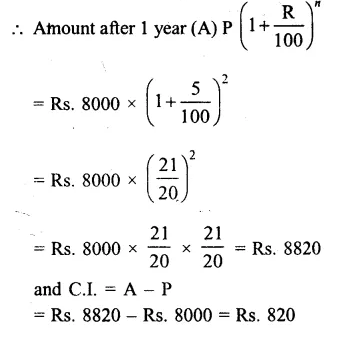Question 2.
Solution:
Principal (P) = Rs. 31250
Rate (R) = 8% p.a. or 4% half yearly
Period (n) = $$1\frac { 1 }{ 2 }$$ years or 3 half years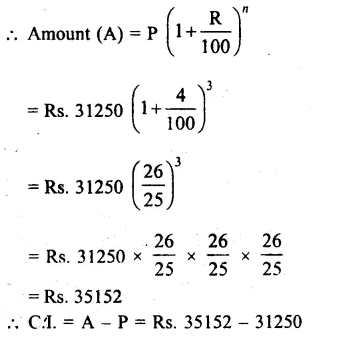= Rs 3902

Question 3.
Solution:
Principal (P) = Rs 12800
Rate (R) = $$7\frac { 1 }{ 2 }$$% p.a. = $$\\ \frac { 15 }{ 2 }$$% half yearly
Period (n) = 1 year or 2 half years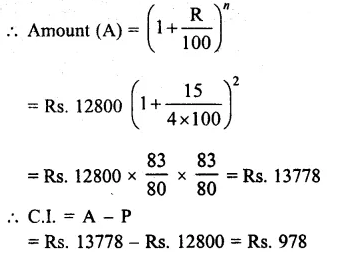Question 4.
Solution:
Principal (P) = Rs. 160000
Rate (R) = 10% p.a. or 5% half yearly
Period (n) = 2 years or 4 half years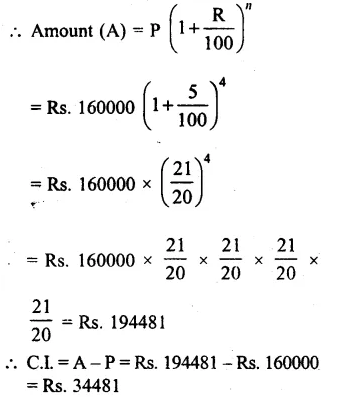Question 5.
Solution:
Principal (P) = Rs. 40960
Rate (R) = $$12\frac { 1 }{ 2 }$$ = $$\\ \frac { 25 }{ 2 }$$% p.a. or $$\\ \frac { 25 }{ 4 }$$ % half yearly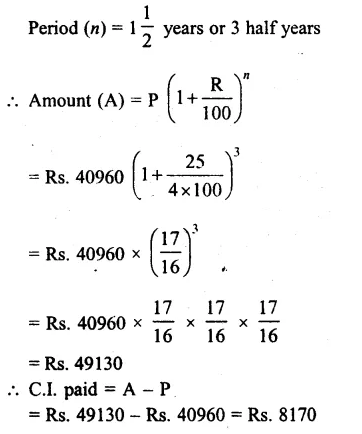Question 6.
Solution:
Loan received for the cost of the house (P) = Rs. 125000
Rate of interest (R) = 12% p.a. or 6% half yearly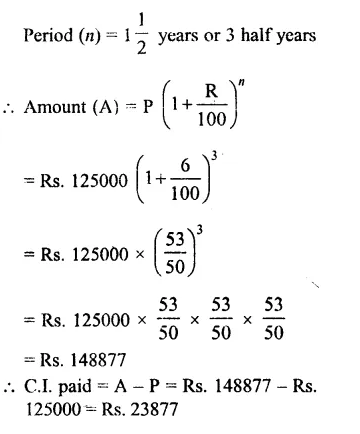Question 7.
Solution:
Amount deposit in the bank = Rs. 20000
Rate of interest (R) = 6% p.a. or 3% half-yearly
Period (n) = 1 year or 2 half years
= $${ \left( 1+\frac { R }{ 100 } \right) }^{ n }$$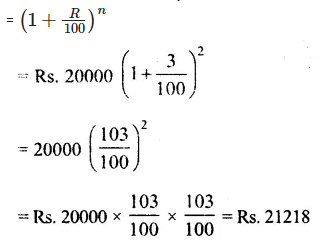Question 8.
Solution:
Amount of loan = Rs. 65536
Rate of interest (R) = 12 $$12\frac { 1 }{ 2 }$$ % = $$\\ \frac { 25 }{ 2 }$$%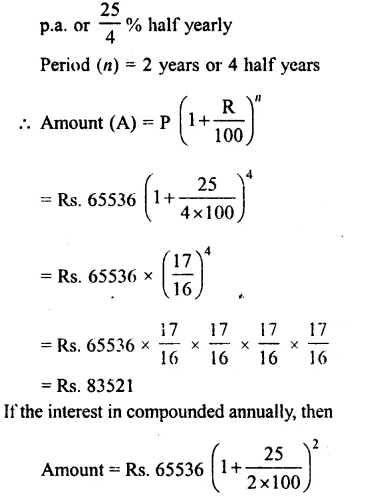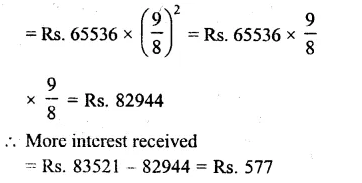Question 9.
Solution:
Amount deposit in the bank (P)
= Rs. 32000
Rate of interest (R) = 5% p.a.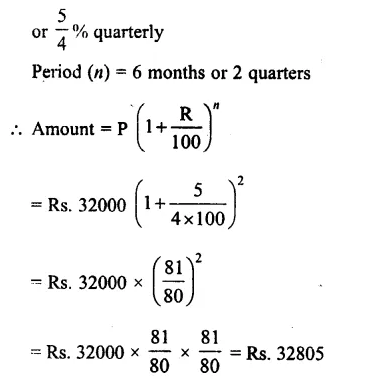Question 10.
Solution:
Amount taken from finance company (P) = Rs. 390625
Rate of interest (R) = 16% p.a.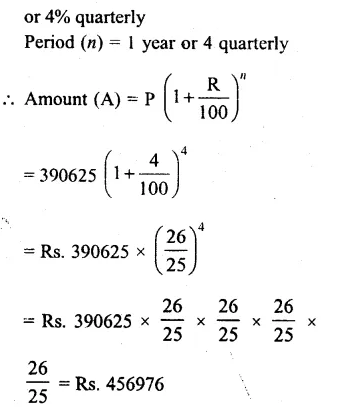Hope given RS Aggarwal Solutions Class 8 Chapter 11 Compound Interest Ex 11C are helpful to complete your math homework.

If you have any doubts, please comment below. Learn Insta try to provide online math tutoring for you.